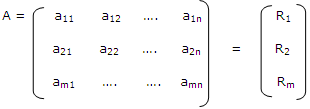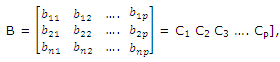Loading

# Describe the row by column matrix

Publish On: 2019-05-08

# Ben joy

Total Post: 542

## Question: Describe the row by column matrix

Reply On: 2013-10-07

# Mjay Jollay

Total Post: 0

## ANS: Describe the row by column matrix

Let A = [aij], B = [bjk].                  I = 1, 2, …., m; j = 1, n; k = 1, …., p

be two matrices of order m × n and n × p respectively.

Let A be written aswhere R, denotes the ith row of the matrix A and it can be regarded as 1 × n matrix. Thus the matrix A can be regarded as an ordered set of rows. Similarly, B can be regarded as the ordered set of columns, i.e.where each Ck k = 1, …. P is a matrix of order n × 1.

#### Submit Your Answer

warning: Please Login To Submit Your Answer
Like Us On Facebook for All Latest Updates